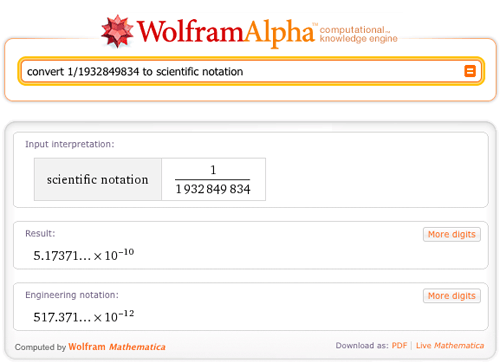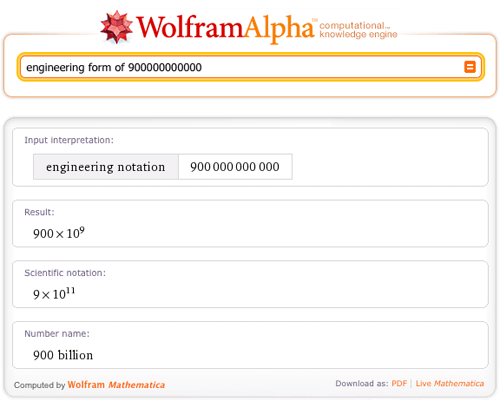# Notating Large and Small Numbers in Wolfram|Alpha

March 23, 2011 —Comments Off

Do you need to work with numbers that are of the magnitude of thousands, millions, or even billions? How about the thousandths, millionths, or billionths? Scientists and engineers need to work with really large and really small numbers every day. Now Wolfram|Alpha can help put all of those large and small numbers into scientific notation. For example, the Earth’s mass is about 5973600000000000000000000 kg, but it is nicely represented in scientific notation as 5.9736×10^24 kg.

One can easily understand the size of a number by looking at the scientific notation rather than counting the number of digits! Wolfram|Alpha can now help by showing any number in scientific notation as well as engineering notation.

Try finding the scientific notation of a really small number, like 1/1932849834, in Wolfram|Alpha.You can even find the scientific notation of a number to a specified precision, like “scientific notation of the millionth prime to precision 5”.Wolfram|Alpha also supports engineering notation. Engineering notation is the same format as scientific notation, but the powers of 10 are multiples of 3.Here you can easily see the difference between scientific notation and engineering notation.

Be sure to consult Wolfram|Alpha to easily understand the magnitude of the next really big or really small number that you stumble across!